# tfsmoment

Conditional spectral moment of the time-frequency distribution of a signal

## Syntax

``momentS = tfsmoment(xt,order)``
``momentS = tfsmoment(x,fs,order)``
``momentS = tfsmoment(x,ts,order) ``
``momentS = tfsmoment(p,fp,tp,order) ``
``momentS = tfsmoment(___,Name,Value)``
``[momentS,t] = tfsmoment(___)``
``tfsmoment(___)``

## Description

Time-frequency moments provide an efficient way to characterize signals whose frequencies change in time (that is, are nonstationary). Such signals can arise from machinery with degraded or failed hardware. Classical Fourier analysis cannot capture the time-varying frequency behavior. Time-frequency distribution generated by short-time Fourier transform (STFT) or other time-frequency analysis techniques can capture the time-varying behavior, but directly treating these distributions as features carries a high computational burden, and potentially introduces unrelated and undesirable feature characteristics. In contrast, distilling the time-frequency distribution results into low-dimension time-frequency moments provides a method for capturing the essential features of the signal in a much smaller data package. Using these moments significantly reduces the computational burden for feature extraction and comparison — a key benefit for real-time operation , .

The Predictive Maintenance Toolbox™ implements the three branches of time-frequency moment:

example

````momentS = tfsmoment(xt,order)` returns the conditional spectral moment of `timetable` `xt` as a `timetable`. The `momentS` variables provide the spectral moments for the orders you specify in `order`. The data in `xt` can be nonuniformly sampled.```

example

````momentS = tfsmoment(x,fs,order)` returns the conditional spectral moment of time-series vector `x`, sampled at rate `Fs`. The moment is returned as a matrix, in which each column represents a spectral moment corresponding each element in `order`. With this syntax, `x` must be uniformly sampled. ```

example

````momentS = tfsmoment(x,ts,order) `returns the conditional spectral moment of `x` sampled at the time instants specified by `ts` in seconds. If `ts` is a scalar `duration`, then `tfsmoment` applies it uniformly to all samples.If `ts` is a vector, then `tfsmoment` applies each element to the corresponding sample in `x`. Use this syntax for nonuniform sampling. ```

example

````momentS = tfsmoment(p,fp,tp,order) `returns the conditional spectral moment of a signal whose power spectrogram is `p`. `fp` contains the frequencies corresponding to the spectral estimate contained in `p`. `tp` contains the vector of time instants corresponding to the centers of the windowed segments used to compute short-time power spectrum estimates. Use this syntax when: You already have the power spectrum or spectrogram you want to use.You want to customize the options for `pspectrum`, rather than accept the default `pspectrum` options that `tfsmoment` applies. Use `pspectrum` first with the options you want, and then use the output `p` as input for `tfsmoment`. This approach also allows you to plot the power spectrogram. ```

example

````momentS = tfsmoment(___,Name,Value)` specifies additional properties using name-value pair arguments. Options include moment centralization and frequency-limit specification.You can use `Name,Value` with any of the input-argument combinations in previous syntaxes.```

example

````[momentS,t] = tfsmoment(___)` returns time vector `t`.You can use `t` with any of the input-argument combinations in previous syntaxes.```

example

````tfsmoment(___)` with no output arguments plots the conditional spectral moment. The plot x-axis is time, and the plot y-axis is the corresponding spectral moment.You can use this syntax with any of the input-argument combinations in previous syntaxes.```

## Examples

collapse all

Plot the second-order conditional spectral moment (variance) of a time series using the plot-only approach and the return-data approach. Visualize the moment differently by plotting the histogram. Compare the moments for data arising from faulty and healthy machine conditions.

This example is adapted from Rolling Element Bearing Fault Diagnosis, which provides a more comprehensive treatment of the data sources and history.

Load the data, which contains vibration measurements for two conditions. `x_inner1` and `sr_inner1` contain the data vector and sample rate for a faulty condition. `x_baseline` and `sr_baseline` contain the data vector and sample rate for a healthy condition.

`load tfmoment_data.mat x_inner1 sr_inner1 x_baseline1 sr_baseline1`

Examine the faulty-condition data. Construct a time vector from the sample rate, and plot the data. Then zoom in to an 0.1-s section so that the behavior can be seen more clearly.

```t_inner1 = (0:length(x_inner1)-1)/sr_inner1; % Construct time vector of [0 1/sr 2/sr ...] matching dimension of x figure plot(t_inner1,x_inner1) title ('Inner1 Signal') hold on xlim([0 0.1]) % Zoom in to an 0.1 s section hold off```The plot shows periodic impulsive variations in the acceleration measurements over time.

Plot the second spectral moment (`order`=2), using the `tfsmoment` syntax with no output arguments.

```order = 2; figure tfsmoment(x_inner1,t_inner1,order) title('Second Spectral Moment of Inner1')```The plot illustrates the changes in the variance of the `x_inner1` spectrum over time. You are limited to this visualization (moment versus time) because `tfsmoment` returned no data. Now use `tfmoment` again to compute the second spectral moment, this time using the syntax that returns both the moment values and the associated time vector. You can use the sample rate directly in the syntax (`sr_inner1`), rather than the time vector you constructed (`t_inner1`).

`[momentS_inner1,t1_inner1] = tfsmoment(x_inner1,sr_inner1,order);`

You can now plot moment versus time as you did before, using `moment_inner1` and `t1_inner1`, with the same result as earlier. But you can also perform additional analysis and visualization of the moment vector, since `tfsmoment `returned the data. A histogram can provide concise information on the signal characteristics.

```figure histogram(momentS_inner1) title('Second Spectral Moment of Inner1')```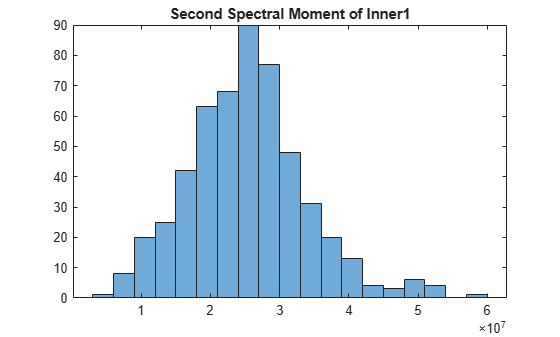On its own, the histogram does not reveal obvious fault information. However, you can compare it to the histogram produced by the healthy-condition data.

First, compare the inner and baseline time series directly using the same time-vector construction for the `baseline1` data as previously for the `inner1` data.

```t_baseline1 = (0:length(x_baseline1)-1)/sr_baseline1; figure plot(t_inner1,x_inner1) hold on plot(t_baseline1,x_baseline1) hold off legend('Faulty Condition','Healthy Condition') title('Vibration versus Time for Faulty and Healthy Conditions')```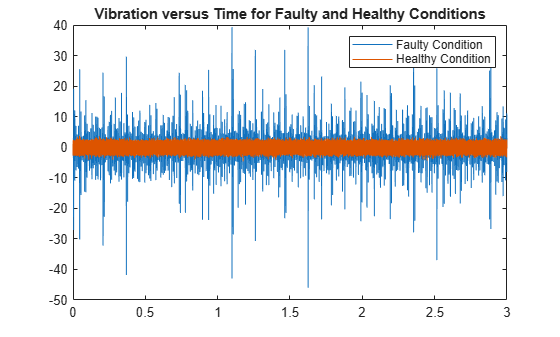Calculate the second spectral moment of the `baseline1` data. Compare the `baseline1` and `inner1` time histories.

```[momentS_baseline1,t1_baseline1] = tfsmoment(x_baseline1,sr_baseline1,2); figure plot(t1_inner1,momentS_inner1) hold on plot(t1_baseline1,momentS_baseline1) hold off legend('Faulty Condition','Healthy Condition') title('Second Spectral Moment versus Time for Faulty and Healthy Conditions')```The moment plot shows behavior different from the earlier vibration plot. The vibration data for the faulty case is much noisier with higher-magnitude spikes than for the healthy case, although both appear to be zero mean. However, the spectral variance (second spectral moment) is significantly lower for the faulty case. The moment of the faulty case is still more noisy than the healthy case.

Plot the histograms.

```figure histogram(momentS_inner1); hold on histogram(momentS_baseline1); hold off legend('Faulty Condition','Healthy Condition') title('Second Spectral Moment for Faulty and Healthy Conditions')```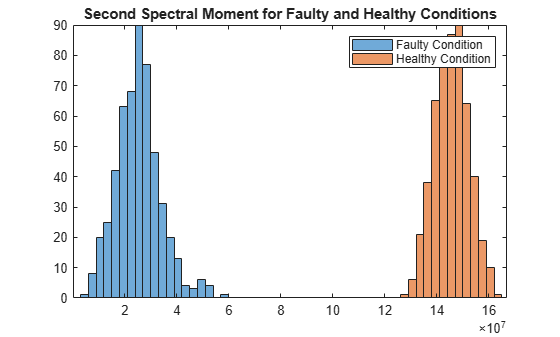The moment behaviors distinguish the faulty condition from the healthy condition in both plots. The histogram provides distinct distribution characteristics — center point along x-axis, spread, and peak histogram bin.

Determine the first four conditional spectral moments of a time-series data set, and extract the moments that you want to visualize with a histogram.

Load the data, which contains vibration measurements (`x_inner1`) and sample rate(`sr_inner1`) for machinery. Then use `tfsmoment` to compute the first four moments. These moments represent the statistical quantities of: 1) Mean; 2) Variance; 3) Skewness; and 4) Kurtosis.

You can specify the moment designators as a vector within the `order` argument.

```load tfmoment_data.mat x_inner1 sr_inner1 momentS_inner1 = tfsmoment(x_inner1,sr_inner1,[1 2 3 4]);```

Compare the dimensions of the input vector and the output matrix.

`xsize = size(x_inner1)`
```xsize = 1×2 146484 1 ```
`msize = size(momentS_inner1)`
```msize = 1×2 524 4 ```

The data vector `x_inner` is considerably longer than the vectors in the moment matrix `momentS_inner1 because the spectrogram computation produces optimally-sized lower-resolution time windows`. ` In this case, tfsmoment` returns a moment matrix containing four columns, one column for each moment order.

Plot the histograms for the third (skewness) and fourth (kurtosis) moments. The third and fourth columns of `momentS_inner1` provide these.

```momentS_3 = momentS_inner1(:,3); momentS_4 = momentS_inner1(:,4); figure histogram(momentS_3) title('Third Spectral Moment (Skewness) of x inner1')``````figure histogram(momentS_4) title('Fourth Spectral Moment (Kurtosis) of x inner1')```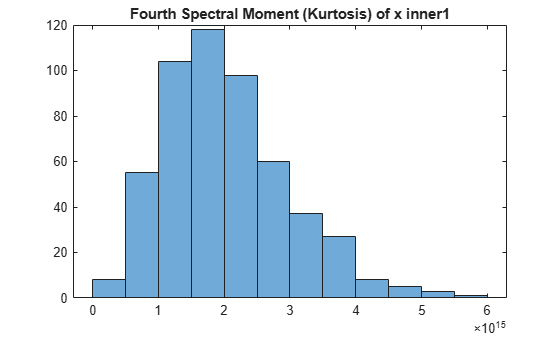The plots are similar, but each has some unique characteristics with respect to number of bins and slope steepness.

By default, `tfsmoment` calls the function `pspectrum` internally to generate the power spectrogram that `tfsmoment` uses for the moment computation. You can also import an existing power spectrogram for `tfsmoment` to use instead. This capability is useful if you already have a power spectrogram as a starting point, or if you want to customize the `pspectrum` options by generating the spectrogram explicitly first.

Input a power spectrogram that has been generated with customized options. Compare the resulting spectral-moment histogram with one that `tfsmoment` generates using its `pspectrum` default options.

Load the data, which includes two power spectrums and the associated frequency and time vectors.

The `p_inner1_def` spectrum was created using the default `pspectrum` options. It is equivalent to what `tfsmoment` computes internally when an input spectrum is not provided in the syntax.

The `p_inner1_MinThr` spectrum was created using the `MinThreshold` `pspectrum` option. This option puts a lower bound on nonzero values to screen out low-level noise. For this example, the threshold was set to screen out noise below the 0.5% level.

```load tfmoment_data.mat p_inner1_def f_p_def t_p_def ... p_inner1_MinThr f_p_MinThr t_p_MinThr load tfmoment_data.mat x_inner1 x_baseline1```

Determine the second spectral moments (variance) for both cases.

```moment_p_def = tfsmoment(p_inner1_def,f_p_def,t_p_def,2); moment_p_MinThr = tfsmoment(p_inner1_MinThr,f_p_MinThr,t_p_MinThr,2);```

Plot the histograms together.

```figure histogram(moment_p_def); hold on histogram(moment_p_MinThr); hold off legend('Moment from Default P','Moment from Customized P') title('Second Spectral Moment for Inner1 from Input Spectrograms')```The histograms have the same overall spread, but the thresholded moment histogram has a higher peak bin at a lower moment magnitude level than the default moment. This example is for illustration purposes only, but does show the impact that preprocessing in the spectrum computation stage can have.

By default, `tfsmoment` centralizes the moment as part of its calculation. That is, it subtracts the sensor-data mean (which is the first moment) from the sensor data as part of the Conditional Spectral Moments. If you wish to preserve the offset, you can set the input argument `Centralize` to f`alse`.

Load the data, which contains vibration measurements x and sample rate sr for machinery. Calculate the 2nd moment (`order` = 2) both with centralization (default), and without centralization (`Centralize` = `false`). Plot the histograms together.

```load tfmoment_data.mat x_inner1 sr_inner1 momentS_centr = tfsmoment(x_inner1,sr_inner1,2); momentS_nocentr = tfsmoment(x_inner1,sr_inner1,2,'Centralize',false); figure histogram(momentS_centr) hold on histogram(momentS_nocentr); hold off legend('Centralized','Noncentralized') title('Second Spectral Moment of x inner1 With and Without Centralization')```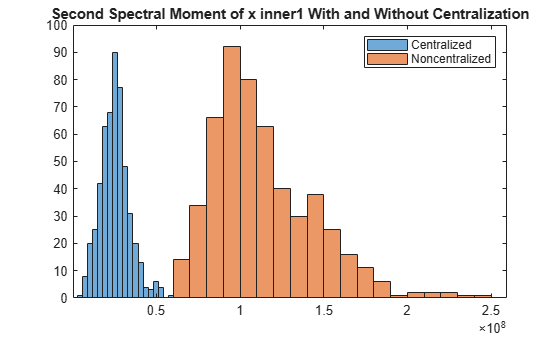The noncentralized distribution is offset to the right.

Real-world measurements often come packaged as part of a time-stamped table that records actual time and readings rather than relative times. You can use the `timetable` format for capturing this data. This example shows how `tfsmoment `operates with a` timetable` input, in contrast to the data vector inputs used for the other `tfsmoment `examples, such as Plot the Conditional Spectral Moment of a Time Series Vector.

Load the data, which consists of a single `timetable` `xt_inner1` containing measurement readings and time information for a piece of machinery. Examine the properties of the `timetable`.

```load tfmoment_tdata.mat xt_inner1; xt_inner1.Properties```
```ans = TimetableProperties with properties: Description: '' UserData: [] DimensionNames: {'Time' 'Variables'} VariableNames: {'x_inner1'} VariableDescriptions: {} VariableUnits: {} VariableContinuity: [] RowTimes: [146484x1 duration] StartTime: 0 sec SampleRate: 4.8828e+04 TimeStep: 2.048e-05 sec CustomProperties: No custom properties are set. Use addprop and rmprop to modify CustomProperties. ```

This table consists of dimensions `Time` and the `Variables`, where the only variable is `x_inner1`.

Find the second and fourth conditional spectral moments for the data in the `timetabl`e. Examine the properties of the resulting moment `timetable`.

```order = [2 4]; momentS_xt_inner1 = tfsmoment(xt_inner1,order); momentS_xt_inner1.Properties```
```ans = TimetableProperties with properties: Description: '' UserData: [] DimensionNames: {'Time' 'Variables'} VariableNames: {'CentralSpectralMoment2' 'CentralSpectralMoment4'} VariableDescriptions: {} VariableUnits: {} VariableContinuity: [] RowTimes: [524x1 duration] StartTime: 0.011725 sec SampleRate: 175.6403 TimeStep: 0.0056935 sec CustomProperties: No custom properties are set. Use addprop and rmprop to modify CustomProperties. ```

The returned `timetable` represents the moments in the variable '`CentralSpectralMoment2`' and '`CentralSpectralMoment4`', providing information not only on what specific moment was calculated, but also on whether it was centralized.

You can access the time and moment information directly from the `timetable` properties. Compute the second and fourth moments. Plot the fourth moment.

```tt_inner1 = momentS_xt_inner1.Time; momentS_inner1_2 = momentS_xt_inner1.CentralSpectralMoment2; momentS_inner1_4 = momentS_xt_inner1.CentralSpectralMoment4; figure plot(tt_inner1,momentS_inner1_4) title('Fourth Spectral Moment of Timetable Data')```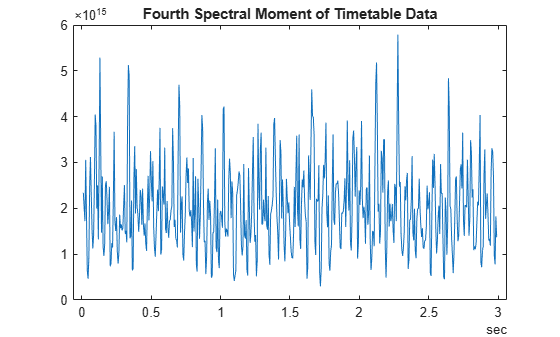As is illustrated in Plot the Conditional Spectral Moment of a Time Series Vector, a histogram is a very useful visualization for moment data. Plot the histogram, directly referencing the `CentralSpectralMoment2` variable property.

```figure histogram(momentS_xt_inner1.CentralSpectralMoment2) title('Second Spectral Moment of xt inner1 Timetable')```## Input Arguments

collapse all

Signal Timetable for which `tfsmoment` returns the moments, specified as a `timetable` that contains a single variable with a single column. `xt` must contain increasing, finite row times. If the timetable has missing or duplicate time points, you can fix it using the tips in Clean Timetable with Missing, Duplicate, or Nonuniform Times. `xt` can be nonuniformly sampled, with the `pspectrum` constraint that the median time interval and the mean time interval must obey.

For an example of timetable input, see Find the Conditional Spectral Moments of Data Measurements in a Timetable

Moment orders to return, specified as one of the following:

• Integer — Compute one moment

• Vector — Compute multiple moments at once.

Example: `momentS = tfsmoment(x,2)` specifies the second-order spectral moment (variance) of the time-frequency distribution of `x`.

Example: `momentS = tfsmoment(x,[1 2 3 4])` specifies the first four moment orders of the time-frequency distribution of `x`.

You can specify any order and number of orders, but low-order moments carry less computational burden and are better suited to real-time applications. The first four moment orders correspond to the statistical moments of a data set:

1. Mean

2. Variance

3. Skewness (degree of asymmetry about the mean)

4. Kurtosis (length of outlier tails in the distribution — a normal distribution has a kurtosis of 3)

For examples, see:

Time-series signal from which `tfsmoment` returns the moments, specified as a vector.

For an example of a time-series input, see Plot the Conditional Spectral Moment of a Time Series Vector

Sample rate of `x`, specified as positive scalar in hertz when `x` is uniformly sampled.

Sample-time values, specified as one of the following:

`ts` can be nonuniform, with the `pspectrum` constraint that the median time interval and the mean time interval must obey:

Power spectrogram or spectrum of a signal, specified as a matrix (spectrogram) or a column vector (spectrum). `p` contains an estimate of the short-term, time-localized power spectrum of a time-series signal. If you specify `p`, `tfsmoment` uses `p` rather than generate its own power spectrogram. For an example, see Use a Customized Power Spectrogram to Compute the Conditional Spectral Moment.

Frequencies for power spectrogram or spectrum `p` when `p` is supplied explicitly to `tfsmoment`, specified as a vector in hertz. The length of `fp` must be equal to the number of rows in `p`.

Time information for power spectrogram or spectrum `p` when `p` is supplied explicitly to `tfsmoment`, specified as one of the following:

• Vector of time points, whose data type can be numeric, `duration`, or `datetime`. The length of vector `tp` must be equal to the number of columns in `p`.

• `duration` scalar that represents the time interval in `p`. The scalar form of `tp` can be used only when `p` is a power spectrogram matrix.

• For the special case where `p` is a column vector (power spectrum), `tp` can be a numeric, `duration`, or `datetime` scalar representing the time point of the spectrum.

### Name-Value Pair Arguments

Specify optional comma-separated pairs of `Name,Value` arguments. `Name` is the argument name and `Value` is the corresponding value. `Name` must appear inside quotes. You can specify several name and value pair arguments in any order as `Name1,Value1,...,NameN,ValueN`.

Example: `'Centralize',false,'FrequencyLimits',[10 100]` computes the noncentralized conditional spectral moment for the portion of the signal ranging from 10 Hz to 100 Hz.

Centralize-moment option, specified as the comma-separated pair consisting of `'Centralize'` and a logical.

• If `Centralize` is `true`, then `tfsmoment` returns the centralized conditional moment by subtracting the conditional mean (which is the first moment) in the computation.

• If `Centralize` is `false`, then `tfsmoment` returns the noncentralized moment, preserving any data offset.

For an example, see Calculate a Conditional Spectral Moment that is not Centralized.

Frequency limits to use, specified as the comma-separated pair consisting of `'FrequencyLimits'` and a two-element vector containing lower and upper bounds f1 and f2 in hertz. This specification allows you to exclude a band of data at either end of the spectral range.

## Output Arguments

collapse all

Conditional spectral moment returned as a `timetable` or a matrix.

Times of moment estimates in seconds. `t` results from the time windowing that the internal spectrogram computation computes. The spectrogram windows require less time resolution than the original sample vector. Therefore, the returned `t` vector is more compact than the input data vectors, as is `momentS`. If time information has been provided by sample rate or sample time,`t` starts from the center of the first time window. If time information has been provided in `duration` or `datetime` format, `t` preserves the start-time offset.

collapse all

### Conditional Spectral Moments

The conditional spectral moments of a nonstationary signal comprise a set of time-varying parameters that characterize the signal spectrum as it evolves in time. They are related to the conditional temporal moments and the joint time-frequency moments. The conditional spectral moment is an integral function of frequency, given time, and marginal distribution. The conditional temporal moment is an integral function of time, given frequency, and marginal distribution. The calculation of the joint time-frequency moment is a double integral that varies both time and frequency , .

Each moment is associated with a specific order, with the first four orders being the statistical properties of 1) mean, 2) variance, 3) skewness, and 4) kurtosis.

`tfsmoment` computes the conditional spectral moments of the time-frequency distribution for a signal `x`, for the orders specified in `order`. The function performs these steps:

1. Compute the spectrogram power spectrum, P(t,f), of the input using the `pspectrum` function and uses it as a time-frequency distribution. If the syntax used supplies an existing P(t,f), then `tfsmoment` uses that instead.

2. Estimate the conditional spectral moment ${〈{\omega }^{m}〉}_{t}$ of the signal using, for the noncentralized case:

`${〈{\omega }^{m}〉}_{t}=\frac{1}{P\left(t\right)}\int {\omega }^{m}P\left(t,\omega \right)d\omega ,$`

where m is the order and P(t) is the marginal distribution.

For the centralized conditional spectral moment ${\mu }_{\omega }^{m}\left(t\right)$, the function uses

`${\mu }_{\omega }^{m}\left(t\right)=\frac{1}{P\left(t\right)}{\int \left(\omega -{〈{\omega }^{1}〉}_{t}\right)}^{m}P\left(t,\omega \right)d\omega .$`

 Loughlin, P. J. "What Are the Time-Frequency Moments of a Signal?" Advanced Signal Processing Algorithms, Architectures, and Implementations XI, SPIE Proceedings. Vol. 4474, November 2001.

 Loughlin, P., F. Cakrak, and L. Cohen. "Conditional Moment Analysis of Transients with Application to Helicopter Fault Data." Mechanical Systems and Signal Processing. Vol 14, Issue 4, 2000, pp. 511–522.